## Jeffrey Aronson: When I Use a Word . . . Medical accents

There is currently a discussion about the differences between related aspects of speech—dialect, accent, and diction.

Dialect is a form of a language that is peculiar to a specific region. The term is usually taken to mean any dialect other than the standard one, such as Standard English.

The IndoEuropean root LEG meant to gather or set in order, to consider or choose, and hence to read or speak. It gave the Greek words λόγος, word, speech, discourse, or reason, and λέγειν, to gather, recount, and speak. These give us the English words logarithm, logic, and logodaedaly; words ending in –ology and –logue (catalogue, decalogue, dialogue, epilogue); lexicon and words ending in –lexia (alexia, dyslexia); and words ending in –lect (analect, intellect, collect, and of course dialect). We get our word dialect from the Greek word διάλεκτος, discourse, debate, argument, conversation, speech, and language.

Linguistics experts have taken the –lect from dialect and coined several other words: acrolect, the most prestigious dialect of a language, basilect, the least prestigious, and mesolect, an intermediate variety, usually having features in common with both the acrolect and the basilect. A cryptolect is a secret language. An idiolect is a dialect peculiar to an individual, which may differ from the varieties of the same dialect used by others. And a sociolect is a dialect used by a particular social group or class. “Genderlect”, The Feminist Dictionary by Cheris Kramarae and Paula A Treichler tells us, is “a notion invented in the early 1970s to suggest overall characterizations of sex differences in speech.” It then says that “[this] portrayal now seems too abstract and overdrawn”, “now” being 1985. But the term is still being written about.

Dialect is typically marked by vocabulary. My Scottish dialect, for instance, includes words such as swithering (in a state of hesitancy or doubt) and dreich (dreary, usually as applied to the weather). It also includes English words used in senses other than are commonly used in England: to go the messages means to do the shopping, and the vegetable known as a swede in England is called a turnip in Scotland.

Dialect and accent usually go together. Scottish dialects, for instance, are typically spoken with a Scottish accent, because they both come from the same place. But the two are not indissolubly linked. And some people are bilingual, able to switch from dialect to Standard English at will.

The IndoEuropean root KAN meant to sing, giving the Latin verb cantare, to sing or to talk about something. This gives us chant and enchanting, cantata, cantor, incantation, descant, recant, and plain cant, the dialect of a class, originally beggars, but now pejoratively extended to any class. Add the Latin prefix ad to cantare and you get accantare, to sing to someone, from which comes accentus, intonation or accent. Accent originally (late 14th century) referred to the way in which something was sung, but it was later applied to the stresses in speech; marks placed above syllables to indicate such stresses were then called accents. Then in the late 16th century it came to mean the way of pronouncing a language.

Although stress, pitch, and rhythm are also involved, the way you pronounce your vowels is a primary characteristic of accent. For example, you can differentiate US and Canadian accents by listening to some of the vowel sounds, for instance in the word “out”; Canadians pronounce it somewhere between the US “owt” and the Scottish “oot”. Australian and New Zealand accents also have different vowel sounds, although the differences are hard to detect.

The foreign accent syndrome is a rare abnormality in which brain damage, typically frontal, or a psychogenic abnormality causes an individual to speak with a foreign accent. The most striking example I have seen was in a young English woman who started speaking with a beautiful French accent after an anaesthetic. Often, however, the accent is not clearly identifiable. We think of accent as something that is conditioned by listening to the people around us, particularly as we grow up. But cases of foreign accent syndrome, rare though they are, show that it’s a lot more complicated than that.

Jeffrey Aronson is a clinical pharmacologist, working in the Centre for Evidence Based Medicine in Oxford’s Nuffield Department of Primary Care Health Sciences. He is also president emeritus of the British Pharmacological Society.

Competing interests: none declared.

 This week’s interesting integer: 341 Geometric numbers Dodecagonal intersections ● Join every pair of points in a dodecagon; there are 341 intersections: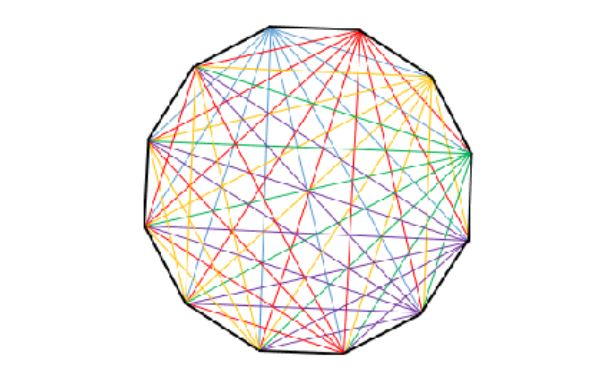Octagonal numbers 341 is the 11th octagonal number, the formula for which is n(3n – 2):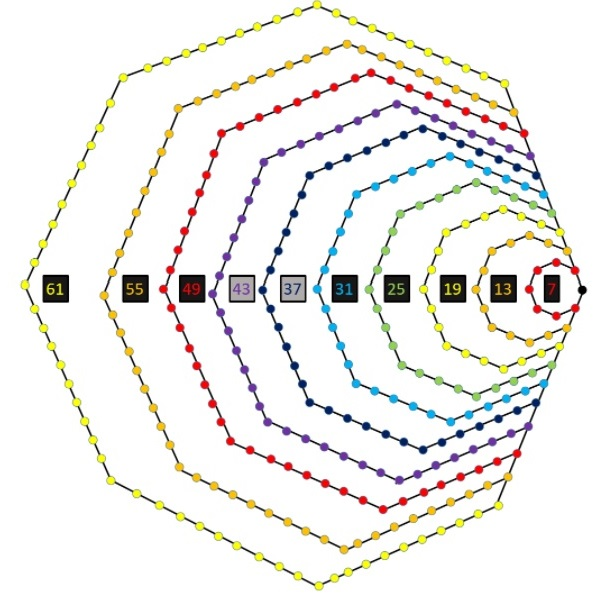● The octagonal numbers also turn up in a hexagonal spiral (the red numbers in the picture below); 341 is in the bottom left-hand corner: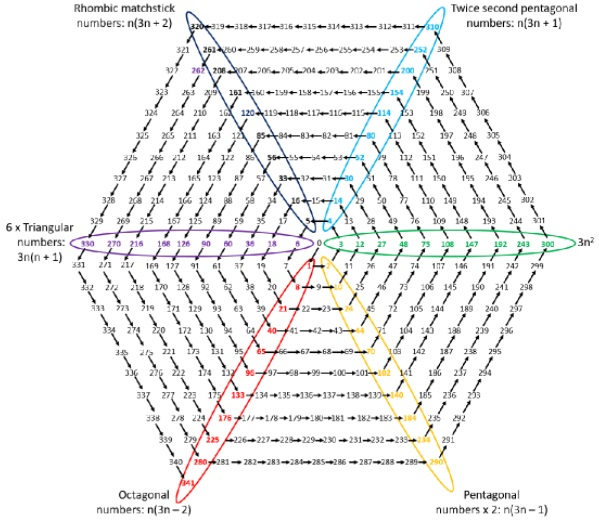Centred cube numbers Start with a single point (black) = 1 point Then construct a cube with two points on each side (red) = 8 points Then construct a cube with three points on each side (blue) = 26 points Now embed black in red (1 + 8 = 9) and embed them in the space in the centre of the blue (1 + 8 + 26 = 35) 1, 9, and 35 are the first three centred cube numbers where n = 0, 1, and 2 in the general formula n3 + (n+1)3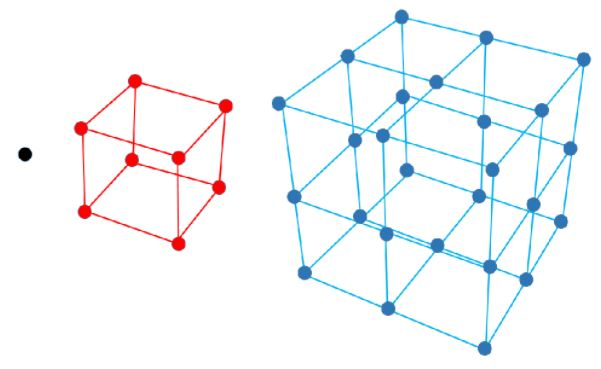Continue constructing bigger cubes a step at a time and embedding them as before; at the  6th stage there will be 341 points in the structure Tiling rectangles There are 5 ways to tile a 3 x 3 rectangle using 1 × 1 squares (red) and 2 × 2 squares (blue):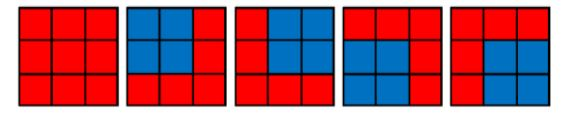There are 11 ways to tile a 3 × 4 rectangle using 1 × 1 and 2 × 2 squares: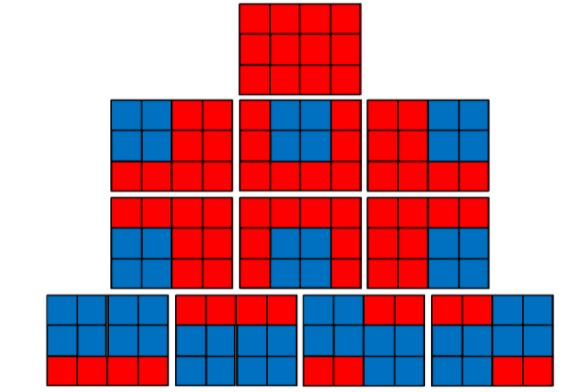There are 21 ways to tile a 3 × 5 rectangle using 1 × 1 and 2 × 2 squares ……. There are 341 ways to tile a 3 × 9 rectangle using 1 × 1 and 2 × 2 squares These are all Jacobsthal numbers—see below Jacobsthal numbers Jacobsthal numbers are from a Fibonacci-like sequence that starts with 0, 1, 1, but instead of adding two numbers to give the next, you add twice the first to the second; the sequence therefore goes 0, 1, 1, 3, 5, 11, 21, 43, 85, 171, 341, … Starting with 1/2, alternately add subtract and add 1/4, 1/8, 1/16, and so on: 1/2 – 1/4 = 1/4 1/2 – 1/4 + 1/8 = 3/8 1/2 – 1/4 + 1/8 – 1/16 = 5/16 1/2 – 1/4 + 1/8 – 1/16 + 1/32 = 11/32 1/2 – 1/4 + 1/8 – 1/16 + 1/32 – 1/64 = 21/64 1/2 – 1/4 + 1/8 – 1/16 + 1/32 – 1/64 + 1/128 = 43/128 ……… 1/2 – 1/4 + 1/8 – 1/16 + 1/32 – 1/64 + 1/128 – 1/256 + 1/512 – 1/1024 = 341/1024 The numerators (red) in these sums are successive Jacobsthal numbers Adding successive pairs of Jacobsthal numbers gives successive powers of 2: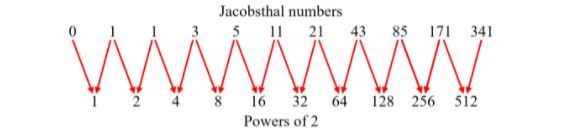Thus, the ratio of successive pairs of Jacobsthal numbers tends to 2 as the series tends to infinity. This property is not found in any other Fibonacci-type sequence in which the nth member (Mn) of the sequence is calculated as Mn = M(n–1) + k × M(n–2) Named numbers 341 is divisible by 31, made from its first and last digits; that makes it a gapful number The divisors of 341 are 1, 11, 31, and 341; the sum of its divisors is 384, the mean of which is 96; since this is an integer, 341 is an arithmetic number 341 is a Curzon number, one for which 2n + 1 is a divisor of 2n + 1; thus, 341 is a divisor of 2341 + 1 Palindromes 341 is palindromic in base 2, base 4, and base 8: 101010101, 11111, and 525 Adding 341 to the product of its digits, 12, gives 353, a palindrome Adding 341 to its inverse, 143, gives 484, a palindrome Divide 341 into two parts, 3 and 41; add them, giving 44, a palindrome Adding 341 to the sum of its prime factors, 42, gives 383, a palindrome Primes and semiprimes 341 is a semiprime = 11 × 31 Its reversal 143 is also a semiprime = 11 × 13, so each is an emirpimes 143 is also a Sophie Germain semiprime, since 2 × 143 + 1 = 287, which is a semiprime = 7 × 41 341 is a base-2 Fermat pseudoprime (or Sarrus number), since 2340 ≡ 1 (mod 341); in other words 2340 when divided by 341 leaves a remainder of 1; 341 is the smallest pseudoprime; these pseudoprimes are named after Pierre Fermat because they are related to his so-called little theorem; Pierre Frédéric Sarrus discovered that 341 is a pseudoprime 3416 + 3415 + 3414 + 3413 + 3412 + 3411 + 3410 is a prime (1,576,891,223,053,147) Pythagorean There are four Pythagorean triples with one short leg equal to 341; the first and last are primitive:  341     420    541 341    1860   1891 341    5280   5291 341   58140  58141 Sums of 341 340 is an electrocardiographic number (see https://blogs.bmj.com/bmj/2021/08/27/jeffrey-aronson-when-i-use-a-word-pharmacographic) Like 340, 341 can be expressed as the sum of different powers of 4: 341 = 40 + 41 + 42 + 43 + 44; that makes it a member of the Moser be Bruijn sequence (see https://blogs.bmj.com/bmj/2021/05/07/jeffrey-aronson-when-i-use-a-word-weighty-words) The divisors of 16 are 1, 2, 4, 8, and 16, and their squares are 40, 41, 42, 43, and 44; thus, 341 is the sum of the squares of the divisors of 16 It is the sum of two consecutive cubes: 53 + 63 It is the sum of consecutive integers in three different ways: 170 + 171 26 + 27 + 28 + 29 + 30 + 31 + 32 + 33 + 34 + 35 + 36 5 + 6 + 7 + 8 + 9 + 10 + 11 + 12 + 13 + 14 + 15 + 16 + 17 + 18 + 19 + 20 + 21 + 22 + 23 + 24 + 25 + 26 It is the sum of 11 consecutive odd integers: 21 + 23 + 25 + 27 + 29 + 31 + 33 + 35 + 37 + 39 + 41 It is the sum of seven consecutive primes: 37 + 41 + 43 + 47 + 53 + 59 + 61 It is the sum of consecutive composite numbers in four different ways: 170 + 171 112 + 114 + 115 45 + 46 + 48 + 49 + 50 + 51 + 52 33 + 34 + 35 + 36 + 38 + 39 + 40 + 42 + 44 It is the sum of three consecutive heptagonal numbers, Hep6 + Hep7 + Hep8: 81 + 112 + 148, where each has the formula n(5n – 3)/2 341 = 44 + 43 + 42 + 41 + 40 Toothpicks Start with a horizontal toothpick (red) and add one at right angles to each end of it (orange); continue for 16 rounds; there will be 341 toothpicks parallel to the first: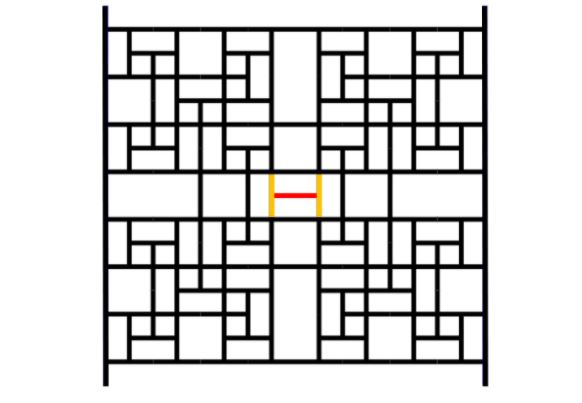Miscellaneous There are 341 odd entries in the first 45 rows of Pascal’s triangle 341 is the 58th term in Aronson’s sequence (see http://neilsloane.com/doc/g4g7.pdf)